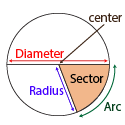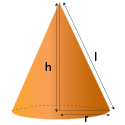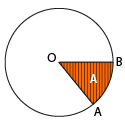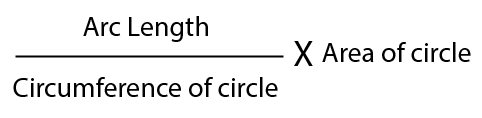Surface area and volume of cone

## Objective

To form a cone from a sector of a circle and to find the formula for its curved surface area.

## What is a Circle?

A circle is a two-dimensional figure formed by a set of points that are at a constant or at a fixed distance (radius) from a fixed point (center) on the plane. The fixed point is called the origin or center of the circle and the fixed distance of the points from the origin is called the radius.

## Parts of CircleIt is also referred to as the perimeter of a circle and can be defined as the distance around the boundary of the circle.

Radius of Circle: Radius is the distance from the center of a circle to any point on its boundary. A circle has many radii as it is the distance from the center and touches the boundary of the circle at various points.

A diameter is a straight line passing through the center that connects two points on the boundary of the circle. We should note that there can be multiple diameters in the circle, but they should:

pass through the center.

be straight lines.

touch the boundary of the circle at two distinct points which lie opposite to each other.

Arc of a Circle: An arc of a circle is referred to as a curve, that is a part or portion of its circumference.

Sector of a Circle: The sector of a circle is defined as the area enclosed by two radii and the corresponding arc in a circle. There are two types of sectors - minor sector, and major sector.

## Circle Formulas

Area of a Circle Formula: The area of a circle refers to the amount of space covered by the circle. It totally depends on the length of its radius → Area = πr2 square units.

Circumference of a Circle Formula: The circumference is the total length of the boundary of a circle → Circumference = 2πr units.

## What is a cone?

• The three elements of the cone are its radius, height, and slant height.
• Radius 'r' is defined as the distance between the center of the circular base to any point on the circumference of the base.
• Height 'h' of the cone is defined as the distance between the apex of the cone to the center of the circular base.
• The slant height 'l' is defined as the distance between the apex of the cone to any point on the circumference of the cone.## Pre-requisite

Sector Area of circle:The sector of a circle OAB is defined as the portion of a circle that is enclosed between its two radii OB, OA and the arc AB adjoining them. The semi-circle is the most common sector of a circle, which represents half a circle.The space enclosed by the sector of a circle is called the area of the sector. For example, a pizza slice is an example of a sector that represents a fraction of a pizza. There are two types of sectors: minor and major sectors. A minor sector is a sector that is less than a semi-circle, whereas, a major sector is a sector greater than a semi-circle.Area of Sector Formula:==The area of a sector circle refers to the amount of defined space covered by the sector of a circle.

Circumference of a Circle: The circumference is the total length of the boundary of a circle → Circumference = 2πr units.